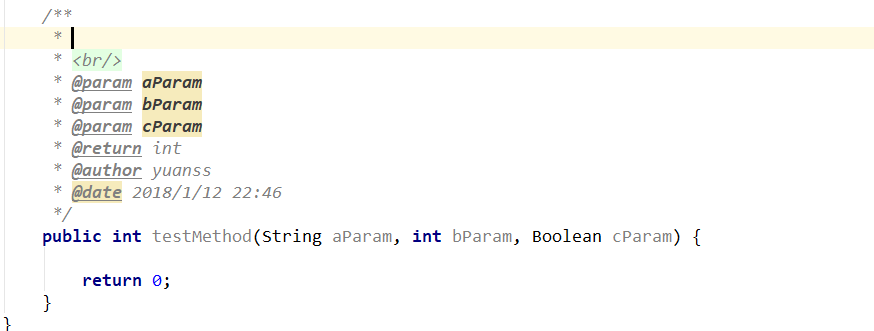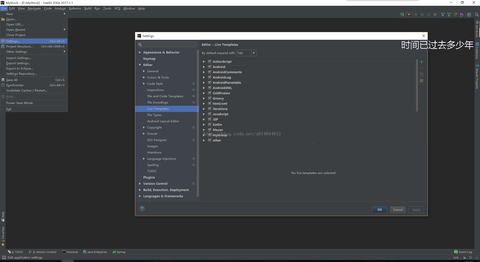IntelliJ IDEA 自定义方法注解模板blog.csdn.net``````/**
*
* <br/>
* @param [aParam, bParam, cParam]
* @return int
* @author yuanss
* @date 2018/1/12 21:41
*/
public int testMethod(String aParam, int bParam, Boolean cParam) {
return 0;
}``````

``````/**
*
* <br/>
* @param aParam
* @param bParam
* @param cParam
* @return int
* @author yuanss
* @date 2018/1/12 21:45
*/
public int testMethod(String aParam, int bParam, Boolean cParam) {
return 0;
}``````

IDEA 中methodParameters(), 貌似是数组的形式(所以是[aParam, bParam, cParam]这样的结构),我看大家都再用一种叫做groovyScript的脚本来分解, 于是我也尝试了,但是呢,copy的脚本又不完美,有点瑕疵.长这个样子:

groovyScript("def result=''; def params=\"\${_1}\".replaceAll('[\\\\[|\\\\]|\\\\s]', '').split(',').toList(); for(i = 0; i < params.size(); i++) {result+=' * @param ' + params[i] + ((i < params.size() - 1) ? '\\n\\b' : '')}; return result", methodParameters())

groovyScript("def result=''; def params=\"\${_1}\".replaceAll('[\\\\[|\\\\]|\\\\s]', '').split(',').toList(); for(i = 0; i < params.size(); i++) {result+=' * @param ' + params[i] + '\\t' + ((i < params.size() - 1) ? '\\n' : '')}; return result", methodParameters())

* ( <------注意这里, 这里巧妙的配合了模板名* )
* \$VAR1\$
* <br/>
\$params\$
* @return \$returns\$
* @author yuanss
* @date \$date\$ \$time\$
*/